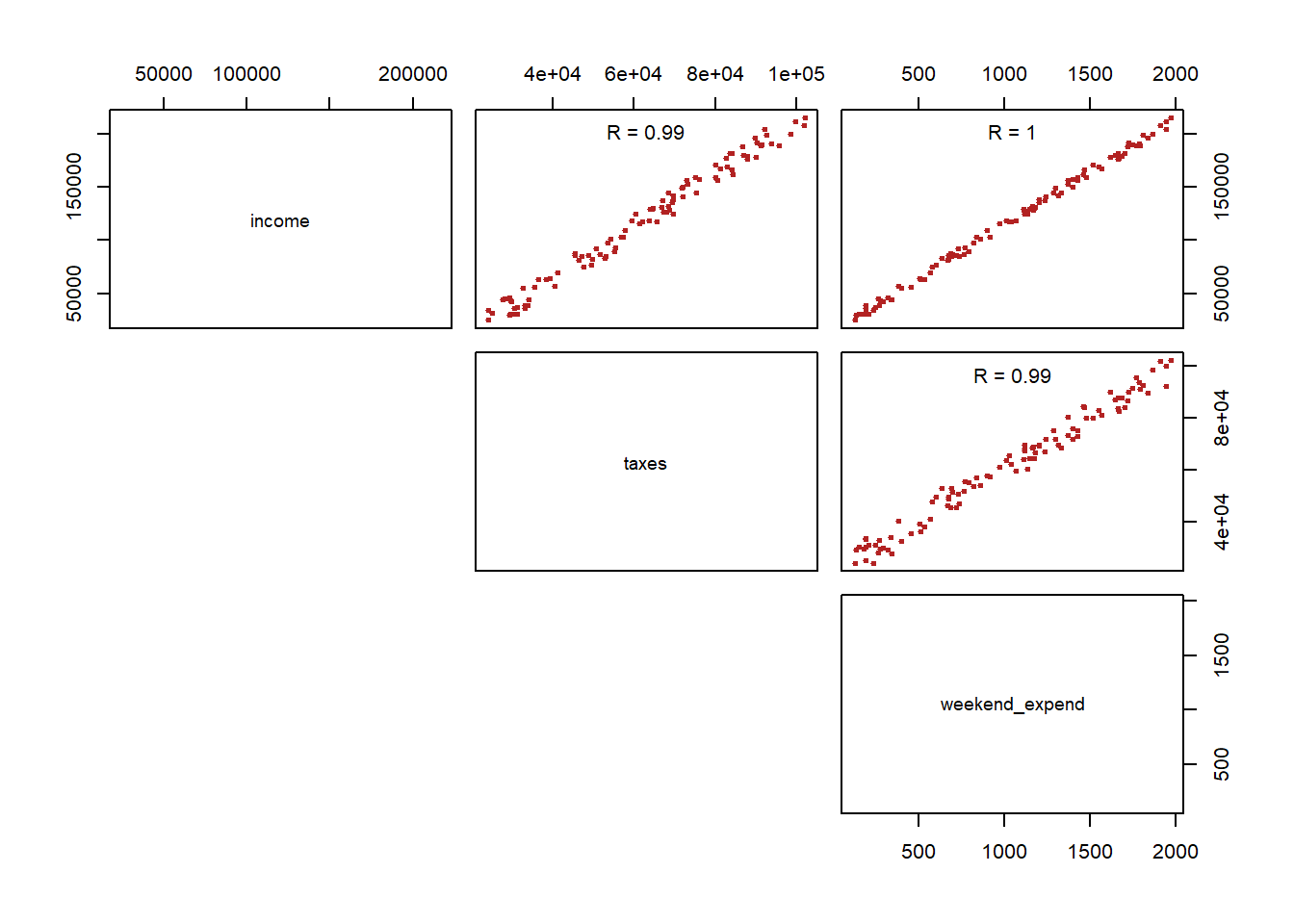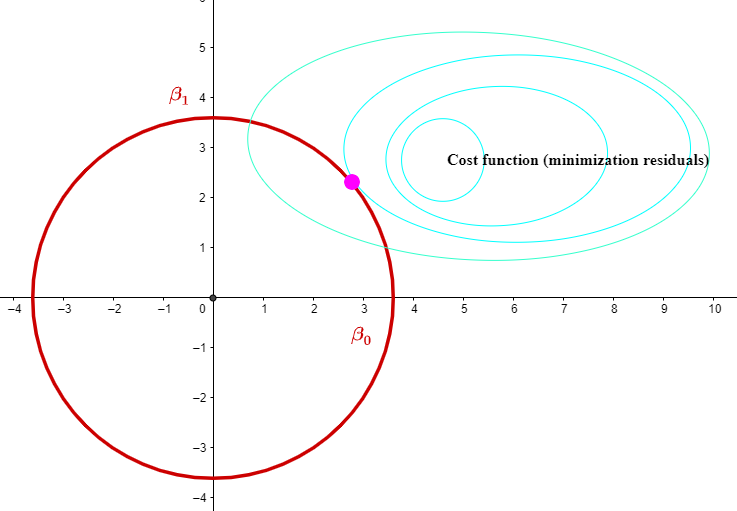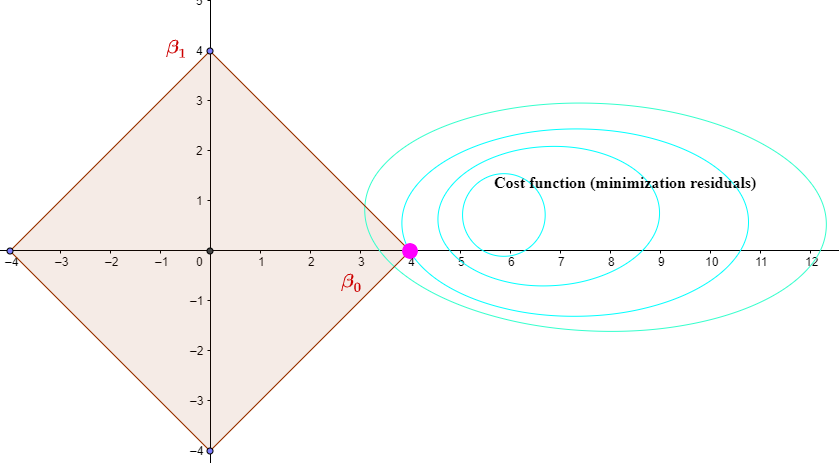#### RIDGE REGRESSION:

OLS minimizes the cost function

$\underset{\vec \beta}{\text{min}}\Vert \vec y- X\vec \beta\Vert^2_2$

which is to say the 2-norm or square residuals. The Gauss-Markov theorem says that these coefficients are BLUE. They are best (B) because they have the smallest variance; and they are also un-biased (U).

This would not work if there was high correlation between the features or explanatory variables, as for example:

$\text{income} \sim \text{income taxes} + \text{money spent on weekends}$

where the two explanatory variables are highly correlated. In this case the calculated coefficients will be highly variable between samples. This is the case where the model matrix is nearly singular, presenting inverse problems.

Let's see what this means with a toy example:

The toy model is trying to predict the yearly income based on paid income taxes and weekend expenses, and all variables are highly correlated:

    set.seed(561)

# The manufacturing of the toy dataset with 100 entries
weekend_expend = runif(100, 100, 2000)
income = weekend_expend * 100 + runif(100, 10000, 20000)
taxes = 0.4 * income + runif(100, 10000, 20000)
df = cbind(income, taxes, weekend_expend)
upper.panel<-function(x, y){
points(x,y, pch=19, col=c("firebrick"), cex=.5)
r <- round(cor(x, y), digits=2)
txt <- paste0("R = ", r)
usr <- par("usr"); on.exit(par(usr))
par(usr = c(0, 1, 0, 1))
text(0.5, 0.9, txt)
}
pairs(df[,1:3], lower.panel = NULL,
upper.panel = upper.panel, cex.labels=.9)options(scipen = 999)

summary(mod <- lm(income ~ weekend_expend + taxes))
##
## Call:
## lm(formula = income ~ weekend_expend + taxes)
##
## Residuals:
##     Min      1Q  Median      3Q     Max
## -5772.9 -1723.2   -73.6  1838.3  6048.8
##
## Coefficients:
##                  Estimate Std. Error t value             Pr(>|t|)
## (Intercept)    5164.91258 1797.73137   2.873              0.00499 **
## weekend_expend   81.57009    3.26427  24.989 < 0.0000000000000002 ***
## taxes             0.46221    0.08139   5.679          0.000000141 ***
## ---
## Signif. codes:  0 '***' 0.001 '**' 0.01 '*' 0.05 '.' 0.1 ' ' 1
##
## Residual standard error: 2627 on 97 degrees of freedom
## Multiple R-squared:  0.9978, Adjusted R-squared:  0.9977
## F-statistic: 2.161e+04 on 2 and 97 DF,  p-value: < 0.00000000000000022
head(A <- model.matrix(mod))
##   (Intercept) weekend_expend    taxes
## 1           1       716.7339 45342.88
## 2           1       837.6407 56916.41
## 3           1       682.5039 45479.96
## 4           1      1396.6189 76087.81
## 5           1       185.5078 33187.67
## 6           1       454.2538 35510.40
(A_tr_A <- t(A) %*% A)
##                (Intercept) weekend_expend        taxes
## (Intercept)          100.0       101686.9      6135129
## weekend_expend    101686.9    133255558.3   7422949538
## taxes            6135128.8   7422949538.2 424423699332
(inv_A_tr_A <- solve(A_tr_A))
##                   (Intercept)    weekend_expend               taxes
## (Intercept)     0.46847390190  0.00076621791905 -0.0000201726356642
## weekend_expend  0.00076621792  0.00000154456888 -0.0000000380895376
## taxes          -0.00002017264 -0.00000003808954  0.0000000009601218
eigen(A_tr_A)$values ##  424553523992.921082 3430995.078305 2.134585 eigen(inv_A_tr_A)$values
##  0.468475155964181489 0.000000291460633770 0.000000000002355416

But this seems to fly on the face of the promised "giant inverse" of $$A^\top A$$ (Prof. Strang's word when explaining penalized regression) in the presence of highly co-linear regressors. Instead the larger eigenvalues seem to go with $$A^\top A$$ as opposed to $$(A^\top A)^{-1}.$$ Professor Strang continues by saying that the matrix $$A$$ is poorly conditioned, "taking vectors almost to zero."

Is it badly conditioned? Let's see the condition number (ratio of largest to smallest eigenvalue):

But first we need to center the mean at zero, and of course, there is no eigenvalues for $$A$$ because it is not square, but we can use the SVD instead:

svd(scale(A,scale = FALSE))$d ##  219214.1817 804.3857 0.0000 in effect, one of its eigenvalues is zero! Let's take a look at the condition number: max(svd(scale(A,scale = FALSE))$d) / min(svd(scale(A,scale = FALSE))$d) ##  Inf Well, $$\inf$$ is pretty large! Same goes for $$A^\top A,$$ also after centering: svd(scale(A_tr_A,scale = FALSE))$d
##  343600135507.86157226562500      2451484.06666654627770
##             0.00000000252724

which outputs an singular value extremely close to zero. Same if we use the eigen() function:

eigen(scale(A_tr_A,scale = FALSE))$values ##  278083427480.8672485351562 1751609.9718418852426 ##  -0.0000001968057 What is the condition number? abs(max(eigen(scale(A_tr_A,scale = FALSE))$values) / min(eigen(scale(A_tr_A,scale = FALSE))$values)) ##  1412984284620866304 Giant! The inverse of $$A^\top A,$$ called as solve(scale(A_tr_A,scale = FALSE)) returns a error: "computationally singular", but if it where numerically computable, it would be pretty large. This is consistent with the fact that when the explanatory variables are highly correlated the variance of the estimated coefficients is large, and related to the inverse of A transpose A: $$\text{Var}(\vec \beta)=\sigma^2 (A^\top A)^{-1}.$$ It is therefore true that when the variables are highly correlated, $$A^\top A$$ will have very low (close to zero) eigenvalues, and $$(A^\top A)^{-1}$$ very high ones. Using ridge regression the estimated coefficients will be biased, although with a lower variance. Ridge is going to control the magnitude of the coefficients. The way this is accomplished is by modifying the cost function as $\underset{\vec \beta}{\text{min}}\Vert \vec y- X\vec \beta\Vert^2_2\text{ such that }\Vert \vec \beta\Vert^2_2\leq c^2$ so in the case of $$2$$ coeffients, we want $$c^2 \leq \beta_0^2 + \beta_1^2,$$ which is a disc of radius $$c:$$Naturally, this should only be remotely applicable when there is more than the intercept and a variable. So it would be a sphere. The estimated values will be at the point of tangential contact of the cost function level curves and the constraint of the parameters (sphere). This is solved with Lagrange multipliers. If $$E=\sum_i \left( y_i - \beta_o-\beta_1\right)^2$$ $F=E + \lambda \left(\beta_0^2 + \beta_1^2 - c^2 \right)$ and we look for $\underset{\vec \lambda,\beta_0,\beta_1}{\text{min}}F$ by trying different $$\lambda$$'s. We could try by differentiating with respect to $$\lambda,\beta_0,\beta_1$$ and setting to zero, but it is usually done numerically. The computation takes into account the R-squared values with different lambdas, which in turn, output different optimal betas. Once we settle on a lambda,$c^2 \$ becomes a constant. So at that point the minimization will be of the function

$F=E + \lambda \left(\beta_0^2 + \beta_1^2 \right)$

or

$\underset{\vec \beta}{\text{min}}\Vert \vec y- X\vec \beta\Vert^2_2 \lambda \Vert\vec \beta \Vert^2_2$

the solution will be

$\vec \beta^R=\left( X^\top X+\lambda I \right)^{-1}X^\top \vec y$

The same with some more math:

In ridge regression we minimize:

$$\bf (y - X\beta)^\prime(y - X\beta) + \lambda \beta^\prime \beta$$

What are the normal equations in this case?

$$\bf (X^\prime X + \lambda I)\beta = X^\prime y$$

Proof:

From this entry, let's consider the square root of $$\lambda$$ to be $$\nu$$, and construct the row augmented model $$[p \times p]$$ matrix, with $$p$$ being the number of columns:

$\bf X_{*} = \pmatrix{X \\ \nu I}$

And the $$\bf y$$ vector augmented by a corresponding $$p$$ number of zeros - $$y_{*}$$.

Now the cost function will be:

$$\bf (y_{*} - X_{*}\beta)^\prime(y_{*} - X_{*}\beta) = (y - X\beta)^\prime(y - X\beta) + \lambda \beta^\prime \beta \tag{1}$$

because there will be $$p$$ additional terms of the form $$(0 - \nu \beta_i)^2 = \lambda \beta_i^2$$

Inspecting the LHS of Eq.1, the normal equations are:

$$\bf (X_{*}^\prime X_{*})\beta = X_{*}^\prime y_{*}\tag{2}$$

Since $$y_{*}$$ just has zeros tagged to the end the RHS of Eq.2 is the same as $$\bf X^\prime y$$, and on the LHS, $$\nu^2 I=\lambda I$$ results in:

$$\bf (X^\prime X + \lambda I)\beta = X^\prime y.$$

By adjoining $$\bf \nu I$$ to $$\bf X$$, thereby lengthening them, we are placing the vectors in avlarger space $$\mathbb R^{n+p}$$ by including $$p$$ "imaginary", mutually orthogonal directions. The first column of $$\bf X$$ is given a small imaginary component of size $$\nu$$, thereby lengthening it and moving it out of the space generated by the original $$p$$ columns. The second, third, ..., $$p^{\text{th}}$$ columns are similarly lengthened and moved out of the original space by the same amount $$\nu$$--but all in different new directions. Consequently, any collinearity present in the original columns will immediately be resolved. Moreover, the larger $$\nu$$ becomes, the more these new vectors approach the individual $$p$$ imaginary directions: they become more and more orthonormal. Consequently, the solution of the normal equations will immediately become possible and it will rapidly become numerically stable as $$\nu$$ increases from zero.

#### LASSO REGRESSION:

On the other hand, in Lasso regression we change the constraint so that some coefficient are nullified. Geometrically, the points jutting out are "hit" more often, and in those corners a number of coefficients will be zero, and the variable associated with it will be nullified (sparsification or feature selection).by minimizing the cost function with the l-$$1$$ norm:

$\underset{\vec \beta}{\text{min}}\Vert \vec y- X\vec \beta\Vert^2_2\text{ such that }\Vert \vec \beta\Vert^1\leq c$

It will be calculated through Lagrange multiplier, but there will not be an explicit formula for Lasso.